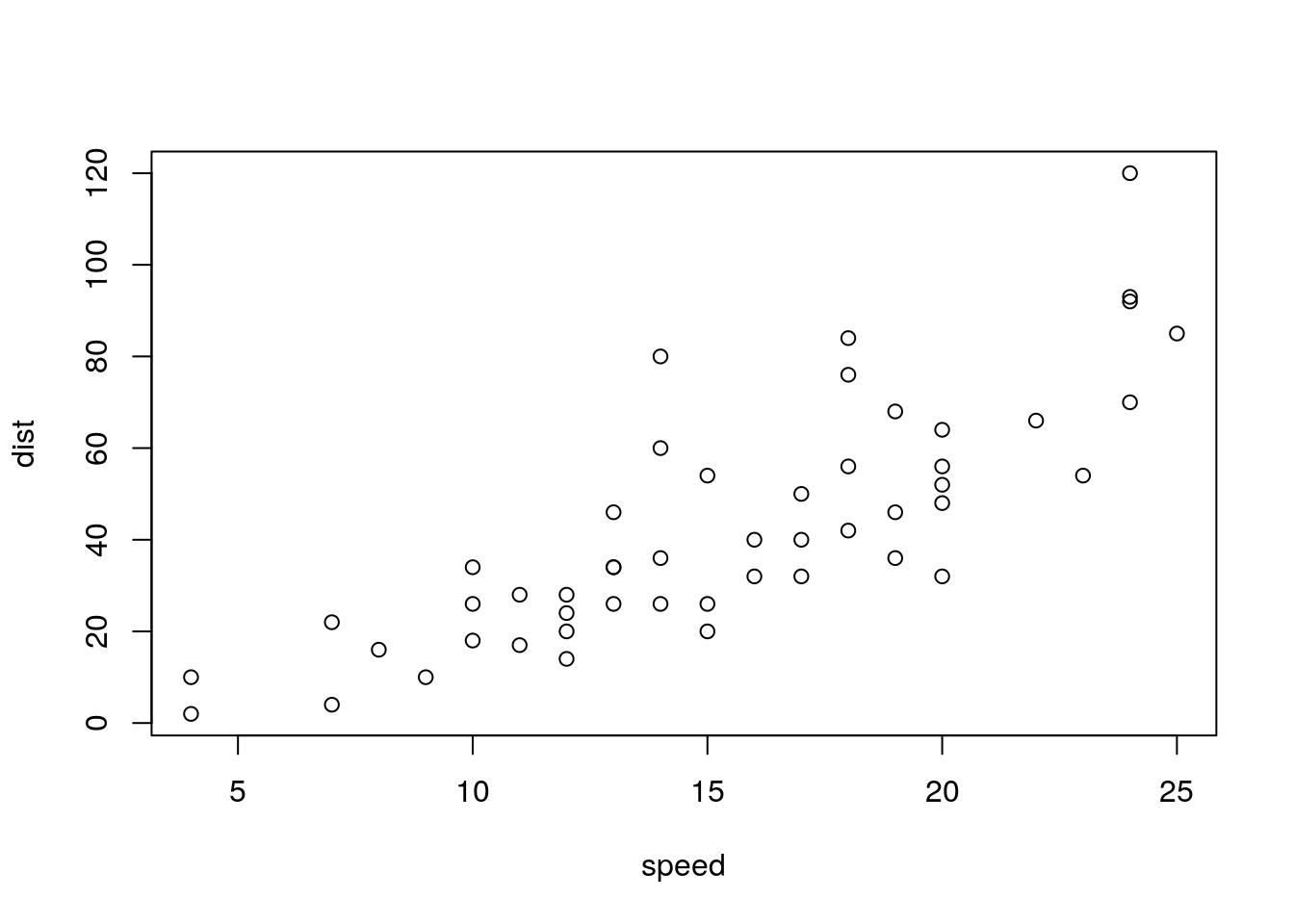# Sample Post

This is an R Markdown document. Markdown is a simple formatting syntax for authoring HTML, PDF, and MS Word documents. For more details on using R Markdown see http://rmarkdown.rstudio.com.

When you click the Knit button a document will be generated that includes both content as well as the output of any embedded R code chunks within the document. You can embed an R code chunk like this:

summary(cars)
##      speed           dist
##  Min.   : 4.0   Min.   :  2.00
##  1st Qu.:12.0   1st Qu.: 26.00
##  Median :15.0   Median : 36.00
##  Mean   :15.4   Mean   : 42.98
##  3rd Qu.:19.0   3rd Qu.: 56.00
##  Max.   :25.0   Max.   :120.00

You can also embed plots, for example:Here is a plot for you.

The border around the graph above is due to custom CSS for this site (see public/css/custom.css). The caption was produced with some HTML:

<p class = "figcaption">Here is a plot for you.</p>

You can write mathematics, of course, but the syntax will be a bit different from R Mardown. Here’s some inline math: $\pi/2 \approx 1.57$. There is displayed math as well:

$\sum_{i=1}^{n} i = \frac{n(n+1)}{2}.$

Here’s the source so you can see how the above worked:

You can write mathematics, of course, but the syntax will be a bit different from R Markdown.  Here's some inline math:  $$\pi/2 \approx 1.57$$.  There is displayed math as well:

$$\sum_{i=1}^{n} i = \frac{n(n+1)}{2}.$$

Here's the source ...

Happy blogging.